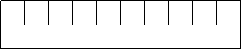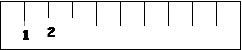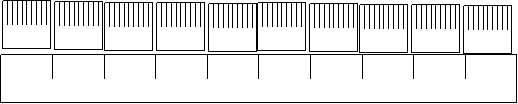# Perplexing Perimeters

Purpose

In this unit the students develop a sense of the size of a centimetre and metre as they construct their own “rulers”.  Students come to recognise the exactness of the measurement needed as they calculate the perimeter of objects.

Achievement Objectives
GM3-1: Use linear scales and whole numbers of metric units for length, area, volume and capacity, weight (mass), angle, temperature, and time.
NA3-1: Use a range of additive and simple multiplicative strategies with whole numbers, fractions, decimals, and percentages.
Specific Learning Outcomes
• Make appropriate measurements accurately using standard units.
• Perform addition calculations to find perimeters of objects.
Description of Mathematics

Perimeter is the distance around the boundary of a shape. Perimeter is a length so is measured in units such as centimetres, metres and kilometres. Perimeters are very important in the construction of enclosures, including fencing, edging and the walls of buildings.

Standard metric units of length are base on the unit of one metre. Prefixes such as centi (one hundredth), milli (one thousandth), and kilo (one thousand) are used to equally partition, or combine, units of one metre, to form new units that are fit for purpose. Length is measured using tools, most frequently rulers or tape measures. Rulers have a scale that shows iterations (copies end on end) of a unit of measure. The marks on the scale indicate the endpoint of units and begin with a baseline of zero. Intervals on a scale can be further subdivided into smaller equal sized units to get greater precision.

The learning opportunities in this unit can be differentiated by providing or removing support to students, by varying the task requirements. Ways to support students include:

• providing equipment, particularly units of measure like 1cm cubes, to help students visualise the units in a scale
• explicit modelling or creating scales, and measuring with them, so students can imitate sound measurement practice

Tasks can be varied in many ways including:

• easing the number calculation demands by choosing objects that have manageable perimeters
• using collaborative grouping so students can support others
• reducing the demands for a product, e.g. less calculations and words, allowing diagrammatic representations.

The contexts for this unit can be adapted to suit the interests and cultural backgrounds of your students. Capitalise on the interests of your students. Perimeters are important in many parts of everyday life. For example, students might be interested in the construction of a fence around a marae, church, or swimming pool. They may be interested in the cost of painting or wallpapering their bedroom. Sport also provides excellent contexts, especially if students are put in the position of marking out playing surfaces. Perimeters are also important for designing borders and for framing art work.

Required Resource Materials
• Strips of heavy card
• Centimetre rulers
• Centimetre cubes
• Rolls of adding machine tape
Activity

#### Session 1

In this session we make our own centimetre rulers and metre tapes so that we can measure the perimeter of objects in the classroom and playground.

1. Gather the class on the mat and tell them that your two pet ants, Anton and Antonia, are having a race. Anton will race around the perimeter of this book and Antonia will race around this book. (Choose two books that are of different shape but similar perimeter). Is the race fair? Is it longer around the perimeter of this book or this book?
Can anyone tell me what perimeter means? (The perimeter of an object or shape is the measure around its edges.)
How could we find out which perimeter is longer?
2. Tell the students that they are going to make their own centimetre rulers.
What is a centimetre?
Can you show me with your fingers how big a centimetre is?
Can you find me something that is one centimetre long? (Centimetre cube, width of a pencil, thickness of a book, width of a fingernail, point to markings on a ruler)
Why are they called centimetres?
Students may know that centi- is the prefix for ‘one hundredth’ so one metre is divided up into 100 centimetres, just like one dollar is divided into 100 cents.
3. Use a metre ruler to explain the relationship between metres and centimetres, if necessary.
4. Give the students a 10 centimetre long strip of card.
How long do you think this strip is?
How could we check? (Use a ruler, line centimetre cubes along the strip.)
5. Can you think of things that are this tall, wide or long?
6. Discuss how they can use the centimetre cube (unit place value block) to make centimetre markings on the strip.
What will the strip look like then?7. Ask: Should we put the marks at the end or in the middle of the centimetres? (The markings are at the end of the unit and those units may need subdivision into smaller units, such as millimetres).
8. Talk about where the number markings are positioned and why they are at the “end” of each centimetre. Talk about where the “0” would go and how this relates to the “0” on commercially produced rulers.
Why is having a zero mark important? (Zero is the conventional baseline to start measuring from)
Why are numbers put on the ruler at all?9. Remind the students that the problem is to compare the perimeters of the two books. Anton and Antonia are awaiting a decision.
How can you keep track of the measurement? (Record and then add the length of the sides later.)
Do you need to measure all of the sides?
What’s the smallest number of sides you need to measure?
10. When the measurements have been made ask the students to sum the side lengths.  This provides a good opportunity to practice and share strategies for adding and multiplying numbers.
How did you combine the numbers?
11. Repeat with the second book and then make the comparison.   This provides further opportunities to share strategies for adding and subtracting numbers.
12. Ask students to select two books from around the room. Can you find two books that are not the same rectangular shape but have very similar perimeters?
13. Draw two or three rectangles that look different but have the same perimeter. Let students investigate the problem in pairs using their personal rulers. After a suitable time share the results.
Is there a way to estimate whether or not two books have the same perimeter before you measure?

#### Session 2

We begin this session by posing a perimeter problem that is “too large” for our 10 cm rulers.  We discuss the need for a larger measuring instrument and then construct and use a 5 metre tape.

1. Gather the class on the mat and tell them that you would like to know if the perimeter of our classroom is larger or smaller than the perimeter of half a netball court.
How could we find out which is larger? Are our centimetre rulers appropriate for this? Why not? What could we use? (metres) How many of our centimetre rulers make a metre? How do you know?
1. Line 10 of the rulers end to end to show a metre.2. Place a strip of adding machine tape, or other long paper strip, alongside the rulers and discuss how it can be used to make markings on the tape.
3. Give each pair of students a 3 metre strip of paper and tell them that they are going to use their 10 cm ruler to create a metre measuring tape.
4. Circulate as the students construct their tape. How many centimetres in a metre? How do you write centimetre? What does this line mean on your tape? Is it at the end of the metre or in the middle? How many centimetres are there in 2 metres? What objects would you choose to measure with the tape rather than the ruler? How accurate is your tape? Why?
5. Remind the students of the question that was posed at the start of the session and ask students to compare the perimeters of the two spaces.
How can you keep track of the measurement? (Record and then add the length of the sides later)
Do you need to measure all of the sides?
How accurate do you need to be? (As the question posed was one of comparison the level of accuracy will depend on how close the perimeters of the two objects are.)
6. If the measurement requires parts of metres to be used then discuss ways of recording these.
1 m 20 cm             120 cm
7. When the measurements have been made ask the students to sum the side lengths.  This provides a good opportunity to practice and share strategies for adding numbers.
How did you combine the numbers?
8. Repeat with the second space and then make the comparison.   This provides further opportunities to share strategies for adding and subtracting numbers.
9. Provide other opportunities to use the metre strip to measure other perimeters:
• What are the perimeters of tables in this room? (Comparing perimeters is very interesting when the tables are different shapes, e.g. circles, trapeziums.
• What is a good perimeter if four students are going to work at a table?
• Does the shape of the table matter?

#### Session 3

In this session the students are involved in a Perimeter Hunt. This involves them finding objects whose perimeter is of a certain length (in either metres or centimetres).

1. Give each pair of students a simple folded booklet into which to record the results of their perimeter hunt.
Ask them to select 10 perimeter ranges to find. Head up each page with a different perimeter range.
• 0 - 10 cm
• 10 - 20 cm
• 20 - 30 cm
• 30 - 40 cm
• 40 - 50 cm
• 50 – 60 cm
• 60 – 70 cm
• 70 – 80 cm
• 90 – 100 cm
• 1 – 2 metres
• 2 – 3 metres
• 5 – 10 metres
• 10 – 20 metres
• Over 20 metres
2. Pose the perimeter hunt challenge: Find objects that have perimeters which match the measure on each page. The pairs should use their handmade measuring tools and record their findings on each page.
3. Draw the object, labelling the measurement for each side, and calculate its perimeter.
4. Discuss methods students use to estimate perimeters before they are measured. Focus on the use of benchmarks like, “I know 10 centimetres is the width of my hand,” or “Two of my steps is about one metre.”
5. Also discuss the impact of the shape on what the perimeter looks like. Why are circles tricky to estimate compared to rectangles? Why do long skinny rectangles look small but have large perimeters?

#### Session 4

In this session the students investigate open ended problems involving perimeter. Students may work in pairs to answer the following problems.

1. How many different shapes can you draw that have a perimeter of 24 centimetres?
2. Explain using words or a diagram why the perimeter of a rectangle can be found by using a combination of addition and multiplication.
3. How could you find the perimeter of a curved shape? (High achieving students might specifically focus on finding the perimeters of circles. That might lead to informal discovery of pi)
4. Imagine you have a rectangle. For one pair of opposite sides, you add one centimetre to each side. You take one centimetre off each of the other two sides. What happens to the perimeter? Why? Does the area stay the same?? Draw some examples to support your answer.

#### Session 5

In this session we compare the objects we found to match the measure in the Perimeter Hunt and discuss the findings in our investigations.

1. Show the students an object and ask them to estimate which “page” of the perimeter book it belongs on.
2. Check the predictions.
What length do you think this side would be?
What do you use to make your estimate?
What about the length of this side? [pointing to another side]
3. Using the agreed estimates ask the students to calculate the perimeter. Share the strategies used for summing the side lengths.
How did you combine the measurements to estimate the perimeter?
The conversation might lead to recording a rule for finding the perimeter of any rectangle.
4. Next ask the students to consider which perimeter was the easiest to find objects for.
Why do you think that [perimeter] was the easiest?
5. Share the objects found for that perimeter length.
6. Ask one pair to read out the side measures for their object while the rest of the students check the calculation mentally. Share the strategies used to sum the numbers mentally.
7. Repeat with other perimeter measures.